﻿ 采煤机牵引部两级行星齿轮传动系统刚柔耦合动力学分析 Analysis for Two-Stage Planetary Gear Transmission System of the Shearer Haulage Unit Based on the Rigid-flexible Coupling Dynamics

Mechanical Engineering and Technology
Vol. 08  No. 05 ( 2019 ), Article ID: 32489 , 10 pages
10.12677/MET.2019.85039

Analysis for Two-Stage Planetary Gear Transmission System of the Shearer Haulage Unit Based on the Rigid-flexible Coupling Dynamics

Decai Yang1,2, Bin Yuan2, Yiliang Wang2*

1Shanxi Coal Mine Machinery Manufacturing Co. Ltd, Taiyuan Shanxi

2Shanxi Key Laboratory of Full Mechanized Coal Mining Equipment, Taiyuan Shanxi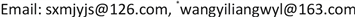Received: Sep. 18th, 2019; accepted: Oct. 4th, 2019; published: Oct. 11th, 2019ABSTRACT

In order to study the driving characteristics of the two-stage planetary gear of the shearer haulage unit and the force of the planet carrier, based on the rigid-flexible coupled multi-body dynamics theory, ANSYS and ADAMS software were used to establish the two-stage planetary gear transmission system rigid-flexible coupling dynamics model. Dynamics simulation of the two-stage planetary gear is carried out based on the Hertz contact theory. The simulation results show that the meshing force of the planetary gear has periodic impact in the time domain and the vibration amplitude has obvious modulation. The response frequency component of the meshing force is composed of a symmetry modulation frequency at which the gear meshing frequency, the gear rotation frequency, and the meshing frequency multiplier are separated by an interval frequency. The stress of the two-stage planet carrier concentrates on the shaft hole of the planet shaft and the root of the output end of the carrier, which is the weak position of the planet carrier. The simulation results not only demonstrate the meshing transmission characteristics of planetary gear are validated but also provide the guidance for studying damage mechanism and fatigue property of the secondary planet carrier.

Keywords:Planetary Gear, Rigid-Flexible Coupled, Meshing Force, Dynamics Simulation

1山西煤矿机械制造股份有限公司，山西 太原

2煤矿综采装备山西省重点实验室，山西 太原1. 引言

2. 刚柔耦合数学模型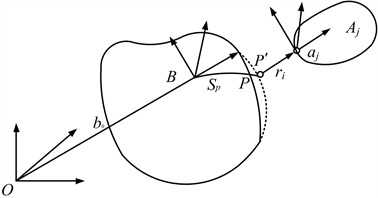Figure 1. Sketch map of the rigid-flexible coupled system of the planetary gear train

${r}_{p}=r+A\left({s}_{p}+{u}_{p}\right)$ (1)

${\stackrel{˙}{r}}_{p}=\stackrel{˙}{r}+\stackrel{˙}{A}\left({s}_{p}+{u}_{p}\right)+A{\stackrel{˙}{u}}_{p}$ (2)

$\left\{\begin{array}{l}\frac{\text{d}}{\text{d}t}\left(\frac{\partial L}{\partial \xi }\right)-\left(\frac{\partial L}{\partial \xi }\right)+\frac{\partial \Gamma }{\partial \xi }+{\left(\frac{\partial \psi }{\partial \xi }\right)}^{\text{T}}\lambda -Q=0\\ \psi =0\end{array}$ (3)

$M\stackrel{¨}{\xi }+\stackrel{˙}{M}\stackrel{¨}{\xi }-\frac{1}{2}{\left(\frac{\partial M}{\partial \xi }\stackrel{˙}{\xi }\right)}^{\text{T}}\stackrel{˙}{\xi }+K\xi +{f}_{g}+D\stackrel{˙}{\xi }+{\left(\frac{\partial \psi }{\partial \xi }\right)}^{\text{T}}\lambda =Q$ (4)

3. 模型建立与动力学仿真

3.1. 刚柔耦合动力学模型建立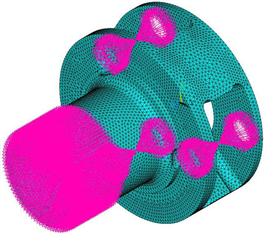Figure 2. Flexible body model of the primary planet carrier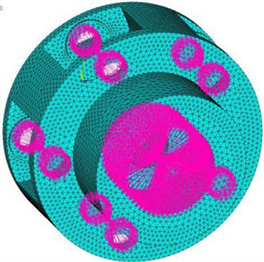Figure 3. Flexible body model of the secondary planetary frame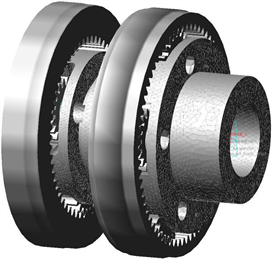Figure 4. Rigid-flexible coupled model of the two- stage planetary system

3.2. 约束与接触参数的施加

$\begin{array}{l}impact\left(x,\stackrel{˙}{x},{x}_{1},K,e,c,d\right)\\ =\left\{\begin{array}{l}\mathrm{max}\left(0,K{\left({x}_{1}-x\right)}^{e}-step\left(x,{x}_{1}-d,c,{x}_{1},0\right)\stackrel{˙}{x}\right)\text{\hspace{0.17em}}\text{\hspace{0.17em}}\text{\hspace{0.17em}}\text{\hspace{0.17em}}x<{x}_{1}\\ 0\text{\hspace{0.17em}}\text{\hspace{0.17em}}\text{\hspace{0.17em}}\text{\hspace{0.17em}}\text{\hspace{0.17em}}\text{\hspace{0.17em}}\text{\hspace{0.17em}}\text{\hspace{0.17em}}\text{\hspace{0.17em}}\text{\hspace{0.17em}}\text{\hspace{0.17em}}\text{\hspace{0.17em}}\text{\hspace{0.17em}}\text{\hspace{0.17em}}\text{\hspace{0.17em}}\text{\hspace{0.17em}}\text{\hspace{0.17em}}\text{\hspace{0.17em}}\text{\hspace{0.17em}}\text{\hspace{0.17em}}\text{\hspace{0.17em}}\text{\hspace{0.17em}}\text{\hspace{0.17em}}\text{\hspace{0.17em}}\text{\hspace{0.17em}}\text{\hspace{0.17em}}\text{\hspace{0.17em}}\text{\hspace{0.17em}}\text{\hspace{0.17em}}\text{\hspace{0.17em}}\text{\hspace{0.17em}}\text{\hspace{0.17em}}\text{\hspace{0.17em}}\text{\hspace{0.17em}}\text{\hspace{0.17em}}\text{\hspace{0.17em}}\text{\hspace{0.17em}}\text{\hspace{0.17em}}\text{\hspace{0.17em}}\text{\hspace{0.17em}}\text{\hspace{0.17em}}\text{\hspace{0.17em}}\text{\hspace{0.17em}}\text{\hspace{0.17em}}\text{\hspace{0.17em}}\text{\hspace{0.17em}}\text{\hspace{0.17em}}\text{\hspace{0.17em}}\text{\hspace{0.17em}}\text{\hspace{0.17em}}\text{\hspace{0.17em}}\text{\hspace{0.17em}}\text{\hspace{0.17em}}\text{\hspace{0.17em}}\text{\hspace{0.17em}}\text{\hspace{0.17em}}\text{\hspace{0.17em}}\text{\hspace{0.17em}}\text{\hspace{0.17em}}\text{\hspace{0.17em}}\text{\hspace{0.17em}}\text{\hspace{0.17em}}x\ge {x}_{1}\end{array}\end{array}$ (5)

$P=K\cdot {\delta }^{3/2}$ (6)

$\left\{\begin{array}{l}K=\frac{4}{3}{R}^{1/2}E\\ \frac{1}{R}=\frac{1}{{R}_{1}}±\frac{1}{{R}_{2}}\\ \frac{1}{E}=\frac{\left(1-{\mu }_{1}^{2}\right)}{{E}_{1}}+\frac{\left(1-{\mu }_{2}^{2}\right)}{{E}_{2}}\end{array}$ (7)Table 1. Meshing stiffness of gears in the planetary system

3.3. 驱动和负载的添加

4. 仿真结果及分析

4.1. 转速特性分析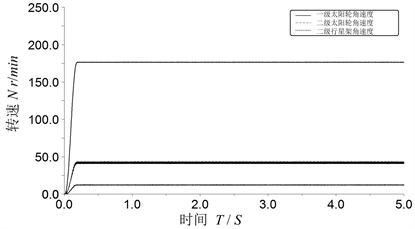Figure 5. Graph of angular velocities

4.2. 行星齿轮啮合频率分析

$\begin{array}{l}{f}_{c}=\frac{{Z}_{s}}{{Z}_{s}+{Z}_{r}}×{f}_{s}\\ {f}_{p}=\left({f}_{s}-{f}_{c}\right)\frac{{Z}_{s}}{{Z}_{p}}-{f}_{c}\end{array}$ (8)

${f}_{m}={f}_{p}\cdot z$ (9)

4.3. 行星齿轮啮合力分析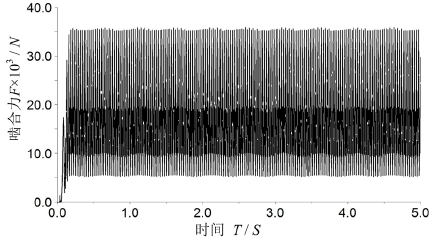(a)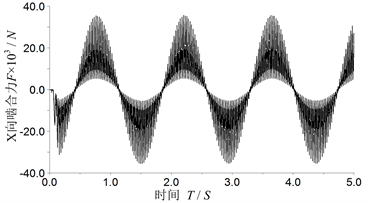(b)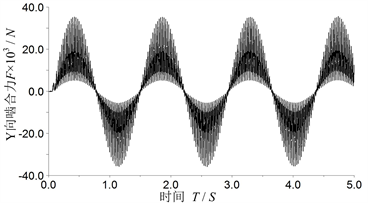(c)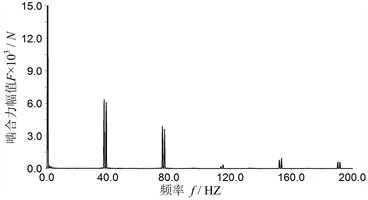(d)

Figure 6. Graph of the engagement forces of the first-order solar wheel. (a) Graph of the engagement forces of the first-order solar wheel; (b) Graph of the engagement force in X direction of the first-order solar wheel; (c) Graph of the engagement force in Y direction of the first-order solar wheel; (d) Frequency spectrum of the engagement force of the first-order solar wheel.(a)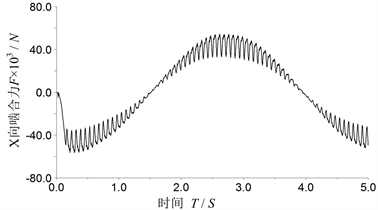(b)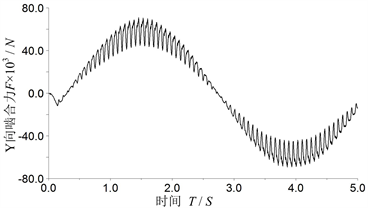(c)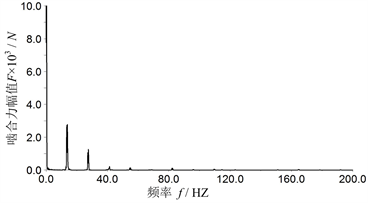(d)

Figure 7. Graph of the engagement forces of the second-order solar wheel. (a) Graph of the engagement forces of the second-order solar wheel; (b) Graph of the engagement force in X direction of the second-order solar wheel; (c) Graph of the engagement force in Y direction of the second-order solar wheel; (d) Frequency spectrum of the engagement force of the second-order solar wheel

4.4. 二级行星架受力分析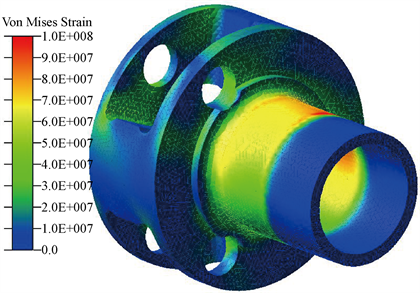(a)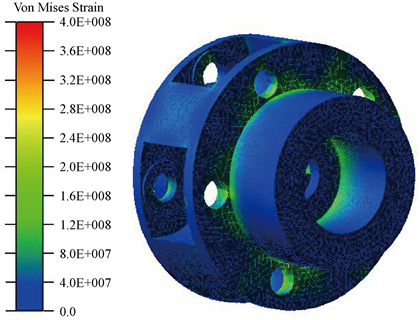(b)

Figure 8. Stress contour of the two planet carriers. (a) Stress contour of the primary planet carrier; (b) Stress contour of the second planet carrier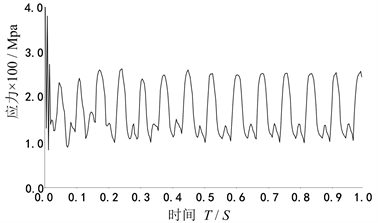Figure 9. Stress at the risk point of the second planet carrier

5. 结论

1) 由于齿轮啮合过程中啮合刚度的改变，各级齿轮啮合力存在着周期性变化的冲击力。啮合力在频域图上的频率成分由齿轮啮合基频、齿轮转频及其啮合倍频两侧以其转频为间隔对称的调制频率带。

2) 从两级行星架应力云图可以看出，二级行星架的应力值比一级行星架大，在工作过程中更容易损坏。

3) 两级行星架都在行星轮轴孔处以及行星架输出轴与前侧板的连接处存在应力集中现象，这些部位为行星架的薄弱环节。

Analysis for Two-Stage Planetary Gear Transmission System of the Shearer Haulage Unit Based on the Rigid-flexible Coupling Dynamics[J]. 机械工程与技术, 2019, 08(05): 327-336. https://doi.org/10.12677/MET.2019.85039

1. 1. 巫世晶, 刘振皓, 潜波, 等. 复合行星齿轮传动系统分岔与混沌特性研究[J]. 华中科技大学学报, 2012, 40(2): 9-13.

2. 2. 石鹏飞, 褚景春, 袁凌, 等. 兆瓦级风电齿轮箱行星架疲劳强度分析[J]. 机械传动, 2017, 41(11): 115-183.

3. 3. 朱卫波, 杨兆建, 王义亮. 采煤机截割部行星齿轮动力学仿真[J]. 煤炭科学技术, 2010, 38(5): 80-82.

4. 4. 张学军, 郭俊超. 基于PRO/E与ADAMS二级行星齿轮传动系统的动力学研究[J]. 组合机床与自动化加工技术, 2015(3): 93-96.

5. 5. 仲昕, 杨汝清, 徐正飞, 等. 多柔体系统动力学建模理论及其应用[J]. 机械科学与技术, 2002, 21(3): 387-389.

6. 6. 李俊虎, 王卫兵, 娄来峰, 等. 双十字轴万向节刚柔耦合动力学研究[J]. 机械传动, 2016, 40(10): 33-37.

7. 7. 李增刚. ADAMS入门详解与实例[M]. 北京: 国防工业出版社, 2010.

8. 8. 黄中华, 张晓健, 周玉军. 渐开线齿轮啮合碰撞力仿真[J]. 中南大学学报, 2011, 42(2): 379-383.

9. 9. 龙凯, 程颖. 齿轮啮合力仿真计算的参数选取研究[J]. 计算机仿真, 2002, 19(6): 87-91.

10. 10. 李润芳, 王建军. 齿轮系统动力学[M]. 北京: 科学技术出版社, 1996.

11. 11. 饶振纲. 行星齿轮传动设计[M]. 北京: 化学工业出版社, 2003.

12. NOTES

*通讯作者。